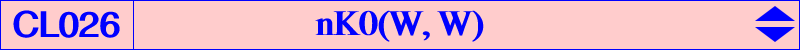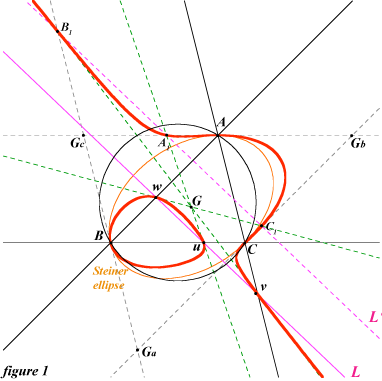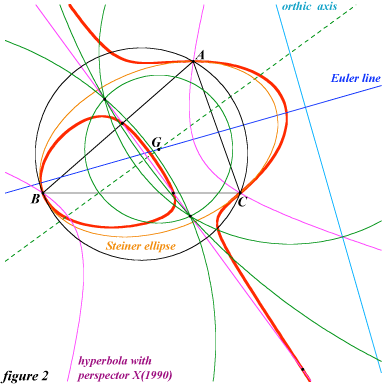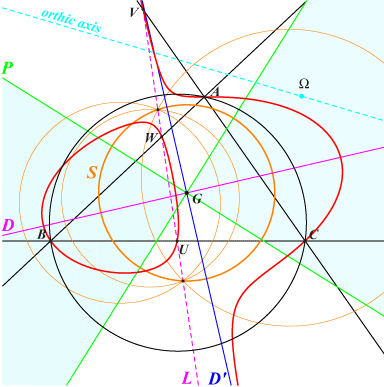A non-pivotal isocubic nK0(W, W) with root and pole W = (p : q : r) is always a K+ with three (not necessarily all real) asymptotes concurring at G (centroid). Its equation is : qr x^2 (y + z) + cyclic = 0. It contains the intersections u, v , w of the trilinear polar L of W with the sidelines of triangle ABC and the tangents at these points concur at G.The tangents at A, B, C are the sidelines of the antimedial triangle GaGbGc and the tangentials A1, B1, C1 of A, B, C are three collinear points on L', the anticomplement of L. Hence, a nK0(W, W) is always tritangent at A, B, C to the Steiner ellipse. See figure 1. Thus, when W lies on the Steiner ellipse, nK0(W, W) must decompose into the Steiner ellipse and a line through G. This is the only case such that the cubic contains G. The asymptotes are all real if and only if W lies inside the Steiner ellipse. This is the case of K024 (isogonal cubic, the only nK60) and K016 (isotomic cubic). This class of cubics contains only one circular cubic, the Sharygin cubic K064 obtained with W = X(1989) which is the orthopivotal cubic O(X523).In general, there is only one point whose polar conic in nK0(W, W) is a circle and it is G, although this circle is degenerated into L and the line at infinity. But when W lies on the orthic axis, the points whose polar conic is a circle belong to a same line D and these polar conics form a pencil of circles whose radical axis is L. This line D is called the circular line of the cubic as in Table 47. It follows that the polar conics of all the points lying on a line different of D have the same directions of axes which are those of the asymptotes of the rectangular circum-hyperbola (h) with perspector W. The general equation of this type of cubic is : ∑ SA x^2 (y + z) / (q-r) = 0, in which the point (p : q : r) is different of G. For example, with W = X(1990), the cubic is nK0(X1990, X1990) and D is the Euler line (figure 2) and with W = X(468), it is the line GK. When W = X(523), X(647), X(650), the line D passes through G and X(525), X(520), X(521) and (h) is the Kiepert, Jerabek, Feuerbach hyperbola respectively. More generally, D is the line passing through G whose infinite point is the barycentric quotient of W and H (orthocenter).A special case Let Ω be the barycentric product of H with one of the infinite points of the Orthocubic K006. The poloconic P of the line at infinity in nK0(Ω, Ω) splits into two perpendicular lines whose bisectors are the circular line D and the orthic line D'. P delimits two regions : • the blue region in which the polar conic of every point is an ellipse, this ellipse becoming a circle for the points on D. In particular, the polar conic of the infinite point of D is the orthoptic circle S of the Steiner inellipse. • the white region in which the polar conic of every point is a hyperbola, this hyperbola becoming rectangular for the points on D'. Recall that every point on P corresponds to a polar conic which is a parabola. At last, the polar conic of G must split into the line at infinity and the trilinear polar L of Ω which is the radical axis of the circles.See the related cubic K600. Other similar cubics in CL044, Q087.